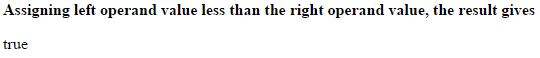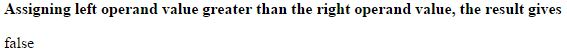# Less than (<) operator in JavaScript

### Less than (<)

Less than operator is an comparison operator which is used to check the value of the left operand is less than the value of the right operand.

If the left operand value is less than the right operand value, it returns 'true'.

The symbolic representation of less than operator is `<`.

### Left operand is less than right operand

```<b>Assigning left operand value less than the right operand value, the result gives</b>
<p id="myId"></p>

<script>
var a = 6;
var b = 10;
var c = (a < b);
document.getElementById("myId").innerHTML = c;
</script>
```

In the above code snippet we have assigned lesser value to the left operand and greater value to the right operand and we used less than operator, so the result gives 'true'.

OUTPUT### Left operand is greater then right operand

```<b>Assigning left operand value greater than the right operand value, the result gives</b>
<p id="myId"></p>

<script>
var a = 10;
var b = 6;
var c = (a < b);
document.getElementById("myId").innerHTML = c;
</script>```

Less than

In the above code snippet we assigned greater value to the left operand and lesser value to the right operand, so the result gives 'false'.

OUTPUTViews: 2272 | Post Order: 172